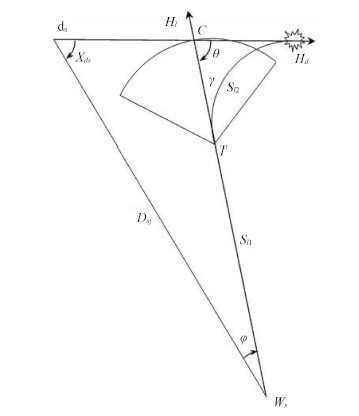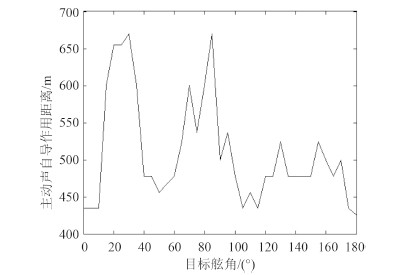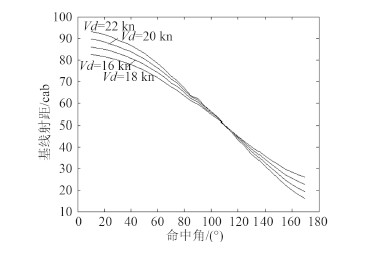﻿ 主动声自导鱼雷极限射距主要影响因素仿真研究
 舰船科学技术2016, Vol. 38Issue (3): 143-146PDF

1. 海军潜艇学院, 山东青岛 266042;
2. 中国人民解放军 91352部队, 山东威海 264200

Research on the simulation of mainly effecting factors about the utmost firing distance of the active acoustic homing torpedo
XU Zhao-peng1, LI Bo1, LI Bin1, WANG Xiang-yang2
1. Navy Submarine Academy, Qingdao 266042, China;
2. No. 91352 Unit of PLA, Weihai 264200, China
Abstract: The appropriate firing distance is one of the most important factors during torpedo attack, the fire distance should be smaller than the utmost firing distance when firing torpedo, so it is necessary to ascertain the firing distance and familiar with the mainly effecting factors which affect the value as well as the effecting rules with the known firing condition. The expression of utmost firing distance is established based on the attacking model of active acoustic homing torpedo; then the mainly effecting factors are gotten. Quantitative research is carried out about the mainly effecting factors by simulation and the effecting law is gotten. Necessary reference is given by this research to the commanding officer during operation, in order to apply this conclusion fluently; so that the hit probability could be improved.
Key words: active acoustic homing torpedo    utmost firing distance    effecting range    relative angle of target
0 引言

1 极限射距及其主要影响因素图 1 主动声自导鱼雷攻击示意图 Fig. 1 Active acoustic homing torpedo attack picture

 ${d_s}C = \frac{{{V_d}}}{{{V_l}}}{S_{l1}}，$ (1)

 ${D_{sj}} \!=\! \sqrt {{{({S_{l1}} \!+ r)}^2}\! + {{(m{S_{l1}})}^2} \!+ 2m{S_{l1}}({S_{l1}} \!+ r)\cos \theta }，$ (2)

 ${D_{sj}} = \sqrt {{S_{l1}}^2(1 + {m^2} + 2m\cos \theta ) + r(r + 2{S_{l1}} + 2m{S_{l1}}\cos \theta )} 。$ (3)

 ${S_{lj}} = {S_{l1}} + {S_{l2}}，$ (4)

 ${S_{l1}} = {S_{lj}} - {S_{l2}}，$ (5)

Sl2 有明确的表达式，即

 ${S_{l2}} = r\frac{{1 - m\cos {X_d}}}{{1 - {m^2}}}。$ (6)

2 主动声自导作用距离

 $TL{\rm{ }} = {\rm{ }}SL{\rm{ }} + {\rm{ }}TS{\rm{ }} - {\rm{ }}NL{\rm{ }} + {\rm{ }}GL{\rm{ }} - {\rm{ }}DT/2。$ (7)

 $TL = SL + TS - RL - DT/2。$ (8)

TL 可表示为扩展损耗 20lnr 和吸收损耗 βr 的和，即

 $TL = 20\ln r + \beta r。$ (9)

 $\begin{array}{*{20}{c}} {\begin{array}{*{20}{c}} {\beta = 1.248\;8 \times {{10}^{ - 5}} - 6.053\;5 \times {{10}^{ - 7}}t + 1.476\;7 \times } \end{array}}\\ {{{10}^{ - 8}}{t^2} - 1.535\;2 \times {{10}^{ - 10}}{t^3} \times 1 - 1.764 \times {{10}^{ - 5}}h{f^2}} \end{array}。$ (10)

 $20\ln r + \beta r = (SL + TS - NL + GL - DT)/2。$ (11)

 $NL = 96\ln {V_l} - 33\ln f - 23.5\ln h。$ (12)

 $TS = 10\ln ({G^2}U)，$ (13)
 $G = \frac{{KABC}}{{2({A^2}{{\cos }^2}{X_d} + {B^2}{{\sin }^2}{X_d})}}，$ (14)
 $\begin{array}{*{20}{c}} {U = \{ 0.251{\mkern 1mu} 635X_d^2 - 0.185{\mkern 1mu} 55{X_d} + }\\ {0.036{\mkern 1mu} 5\sin [3({X_d} + 0.174{\mkern 1mu} 537)] + }\\ {\quad 0.015X_d^2\sin (9{X_d}/2){\} ^{ - 1}}} \end{array}。$ (15)

3 仿真及分析图 2 主动声自导鱼雷作用距离 Fig. 2 The effecting range of active acoustic homing torpedo

r 的求解过程可看出其值与目标速度没有联系。图 2r 明显具有离散性，其与潜艇目标强度所具有的离散性相一致。即潜艇某一方向上的强度大，则在该方向上鱼雷主动作用距离就大。正横方向上，目标强度大由艇壳的镜反射引起；首尾方向上，目标强度小由艇壳和尾流的遮蔽效应引起；在艇首 30°附近强度大，估计由舱室结构的内反射产生。图 3 主动声自导鱼雷极限射距 Fig. 3 The utmost firing distance of active acoustic homing torpedo

4 结语

  赵加余. 潜艇攻击常识[M]. 青岛:海军潜艇学院, 2003.  毛俊超, 邱华, 孙华春. 声自导鱼雷追踪导引弹道分析[J]. 舰船科学技术, 2011, 33(4):123-125.MAO Jun-chao, QIU Hua, SUN Hua-chun. The pursuit-guidance trajectory analysis for acoustic homing torpedo[J]. Ship Science and Technology, 2011, 33(4):123-125.  赵加余. 潜艇攻击[M]. 青岛:海军潜艇学院, 2003.  何心怡, 钱东, 王光宇, 等. 水文条件对鱼雷声自导作用距离的影响[J]. 鱼雷技术, 2007, 15(5):33-36.HE Xin-yi, QIAN Dong, WANG Guang-yu, et al. Influence of water conditions on torpedo acoustic homing range[J]. Torpedo Technology, 2007, 15(5):33-36.  初磊, 楼晓平, 李世令, 等. 鱼雷声自导作用距离影响因素仿真分析[J]. 弹箭与制导学报, 2010, 30(1):68-71.CHU Lei, LOU Xiao-ping, LI Shi-ling, et al. Analysis of influence parameter to acoustically guided torpedo operating distance[J]. Journal of Projectiles, Rockets, Missiles and Guidance, 2010, 30(1):68-71.  刘伯胜, 雷家煜. 水声学原理[M]. 哈尔滨:哈尔滨工程大学出版社, 2006.